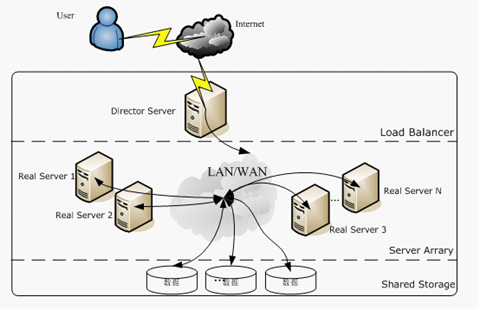数据可视化之箱形图

箱形图简介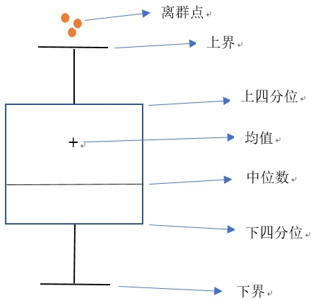• 第一四分位数（$Q_1$）：也称下四分位数（Lower Quartile），等于该样本中所有数值由小到大排列后第25%的数字。
• 第二四分位数（$Q_2$）：也称中位数（Middle Quartile or Median），等于该样本中所有数值由小到大排列后第50%的数字。
• 第三四分位数（$Q_3$）：也称上四分位数（Upper Quartile），等于该样本中所有数值由小到大排列后第75%的数字。

$Q_3$和$Q_1$的差距称为四分位距（InterQuartile Range， IQR）：

$${\Delta}Q = Q_3-Q_1$$

$$\text{Lower Whisker} = Q_1 – 1.5{\Delta}Q$$

$$\text{Upper Whisker} = Q_3 + 1.5{\Delta}Q$$

• 内限：$Q_1 – 1.5{\Delta}Q$和$Q_3 + 1.5{\Delta}Q$
• 外限：$Q_1 – 3{\Delta}Q$和$Q_3 + 3{\Delta}Q$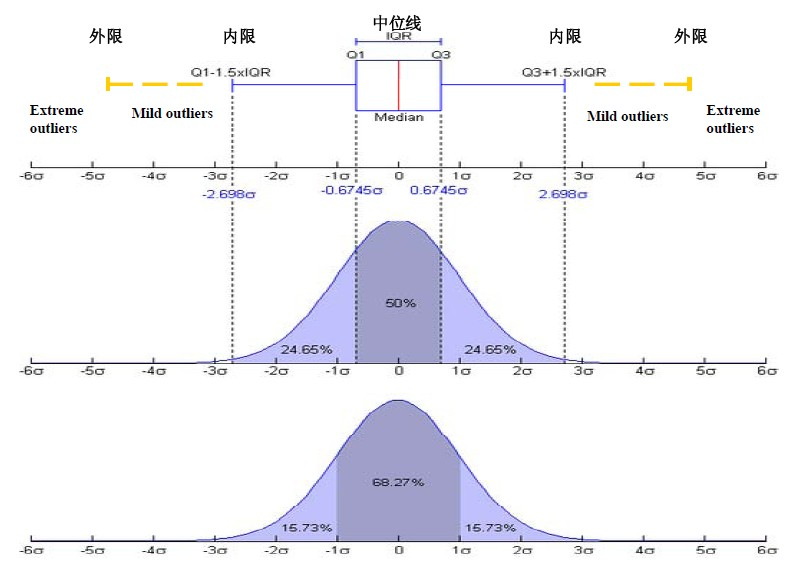箱形图的Python实现

matplotlib.pyplot.boxplot()

matplotlib.pyplot.boxplot(x, notch=None, sym=None, vert=None, whis=None, positions=None, widths=None, patch_artist=None, bootstrap=None, usermedians=None, conf_intervals=None, meanline=None, showmeans=None, showcaps=None, showbox=None, showfliers=None, boxprops=None, labels=None, flierprops=None, medianprops=None, meanprops=None, capprops=None, whiskerprops=None, manage_ticks=True, autorange=False, zorder=None, *, data=None)

• x： 数组或者向量序列，指定要绘制箱线图的数据
• notch：是否是凹口箱线图，默认为否。凹口表示中值附近的置信区间
• sym：指定异常点的形状，默认是+号显示
• vert：是否需要将箱线图垂直摆放，默认垂直摆放
• whis：指定上下须跟上下四分位的距离，默认是5倍四分位差
• positions：指定箱线图的位置，默认为[0,1,2…]
• widths：设置每个框的宽度，标量或者序列，默认5
• patch_artist：默认是用二维线性绘制（Line2D artist）
• bootstrap：是否为凹口位置的中位数设置置信区间
• usermedians：设置每个元素的中位数，默认由matplotlib计算
• conf_intervals：置信区间
• meanline：是否用线的形式表示均值，默认用点来表示
• showmeans：是否显示均值，默认不显示
• showcaps：是否显示箱线图顶端和末端的两条线，默认显示
• showbox：是否显示箱线图的箱体，默认显示
• showfliers：是否显示异常值，默认显示
• boxprops：设置箱体的属性，如边框色，填充色等
• labels：为箱线图添加标签，类似于图例的作用
• filerprops：设置异常值的属性，如异常点的形状、大小、填充色等
• medianprops：设置中位数的属性，如线的类型、粗细等
• meanprops：设置均值的属性，如点的大小、颜色等
• capprops：设置箱线图顶端和末端线条的属性，如颜色、粗细等
• whiskerprops：设置须的属性，如颜色、粗细、线的类型等
• manage_ticks：调整tick的位置，默认True
• autorange：是否用线的形式来表示均值，默认是点
• zorder：设置箱线图的zorder

import matplotlib.pyplot as plt
import pandas as pd

# 添加成绩表
plt.style.use("ggplot")
plt.rcParams['axes.unicode_minus'] = False
plt.rcParams['font.sans-serif'] = ['SimHei']

# 新建一个空的DataFrame
df = pd.DataFrame()

df["英语"] = [76, 90, 97, 71, 70, 93, 86, 83, 78, 85, 81]
df["经济数学"] = [65, 95, 51, 74, 78, 63, 91, 82, 75, 71, 55]
df["西方经济学"] = [93, 81, 76, 88, 66, 79, 83, 92, 78, 86, 78]
df["计算机应用基础"] = [85, 78, 81, 95, 70, 67, 82, 72, 80, 81, 77]

plt.boxplot(x=df.values, labels=df.columns, whis=1.5)
plt.show()

pandas.DataFrame.boxplot()

DataFrame.boxplot(column=None, by=None, ax=None, fontsize=None, rot=0, grid=True, figsize=None, layout=None, return_type=None, **kwds)

• column：默认为None，输入为str 或由str构成的list,其作用是指定要进行箱型图分析的列
• by：默认为None，str or array-like，其作用为pandas的group by，通过指定by=‘columns’，可进行多组合箱型图分析
• ax：matplotlib.axes.Axes的对象，没有太大作用
• fontsize：箱型图坐标轴字体大小
• rot：箱型图坐标轴旋转角度
• grid：箱型图网格线是否显示
• figsize：箱型图窗口尺寸大小
• layout：必须配合by 一起使用，类似于subplot 的画布分区域功能
• return_type：指定返回对象的类型，默认为None,可输入的参数为‘axes’,‘dict’,‘both’，当与by一起使用是，返回的对象为Series或array(for return_type = None)
• **kwds：All other plotting keyword arguments to be passed to matplotlib.pyplot.boxplot().

import matplotlib.pyplot as plt
import pandas as pd

# 添加成绩表
plt.style.use("ggplot")
plt.rcParams['axes.unicode_minus'] = False
plt.rcParams['font.sans-serif'] = ['SimHei']

# 新建一个空的DataFrame
df = pd.DataFrame()

df["英语"] = [76, 90, 97, 71, 70, 93, 86, 83, 78, 85, 81]
df["经济数学"] = [65, 95, 51, 74, 78, 63, 91, 82, 75, 71, 55]
df["西方经济学"] = [93, 81, 76, 88, 66, 79, 83, 92, 78, 86, 78]
df["计算机应用基础"] = [85, 78, 81, 95, 70, 67, 82, 72, 80, 81, 77]

df.boxplot()
plt.show()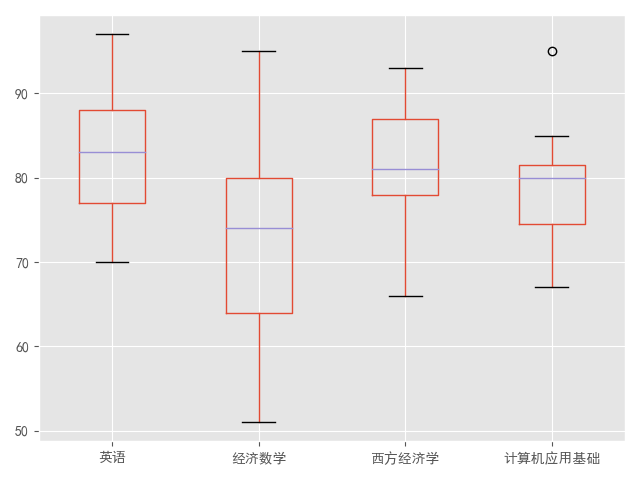seaborn.boxplot()

seaborn.boxplot(x=None, y=None, hue=None, data=None, order=None, hue_order=None, orient=None, color=None, palette=None, saturation=0.75, width=0.8, dodge=True, fliersize=5, linewidth=None, whis=1.5, ax=None, **kwargs)

• x：指定x轴的分类名称
• y：指定y轴的分类名称
• hue：指定第二次分类的数据类别(用颜色区分)
• data：数组或数组列表
• order：显式指定指定第二次分类顺序
• hue_order：显式指定分类顺序
• orient：设置图的绘制方向(垂直或水平)，v或者h
• color：matplotlib 颜色
• palette：调色板名称，list类别或者字典，用于对数据不同分类进行颜色区别
• saturation：控制用于绘制颜色的原始饱和度的比例。通常大幅填充在轻微不饱和的颜色下看起来更好，如果您希望绘图颜色与输入颜色规格完美匹配可将其设置为1。
• width：不使用色调嵌套时完整元素的宽度，或主要分组变量一个级别的所有元素的宽度。
• dodge：若设置为True则沿着分类轴，将数据分离出来成为不同色调级别的条带，否则，每个级别的点将相互叠加
• fliersize：用于表示异常值观察的标记的大小。
• linewidth：设置构图元素的线宽度
• whis：控制在超过高低四分位数时 IQR 的比例，因此需要延长绘制的触须线段。超出此范围的点将被识别为异常值。
• ax：matplotlib 轴，可选
• **kwargs：pyplot.boxplot()中的其他参数

import matplotlib.pyplot as plt
import pandas as pd
import seaborn as sns

# 添加成绩表
plt.style.use("ggplot")
plt.rcParams['axes.unicode_minus'] = False
plt.rcParams['font.sans-serif'] = ['SimHei']

# 新建一个空的DataFrame
df = pd.DataFrame()

df["英语"] = [76, 90, 97, 71, 70, 93, 86, 83, 78, 85, 81]
df["经济数学"] = [65, 95, 51, 74, 78, 63, 91, 82, 75, 71, 55]
df["西方经济学"] = [93, 81, 76, 88, 66, 79, 83, 92, 78, 86, 78]
df["计算机应用基础"] = [85, 78, 81, 95, 70, 67, 82, 72, 80, 81, 77]

sns.boxplot(data=df)
plt.show()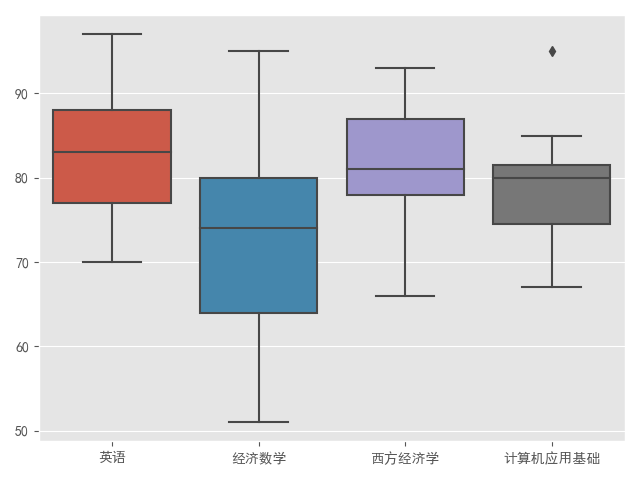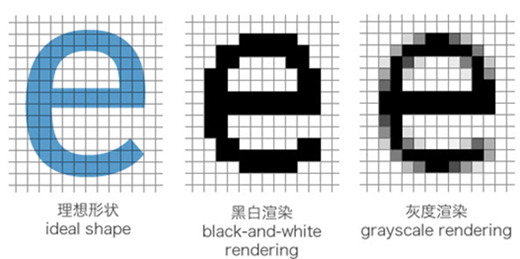网站与APP开发中的字体设置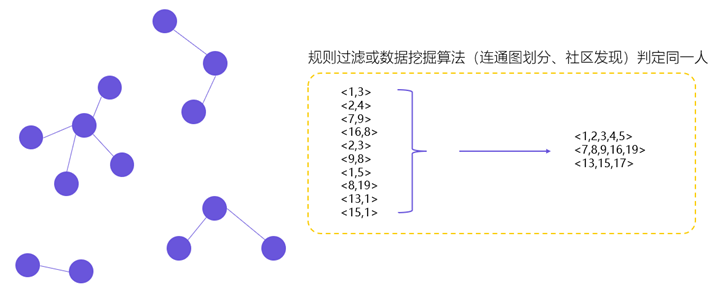用户体系搭建之ID-Mapping